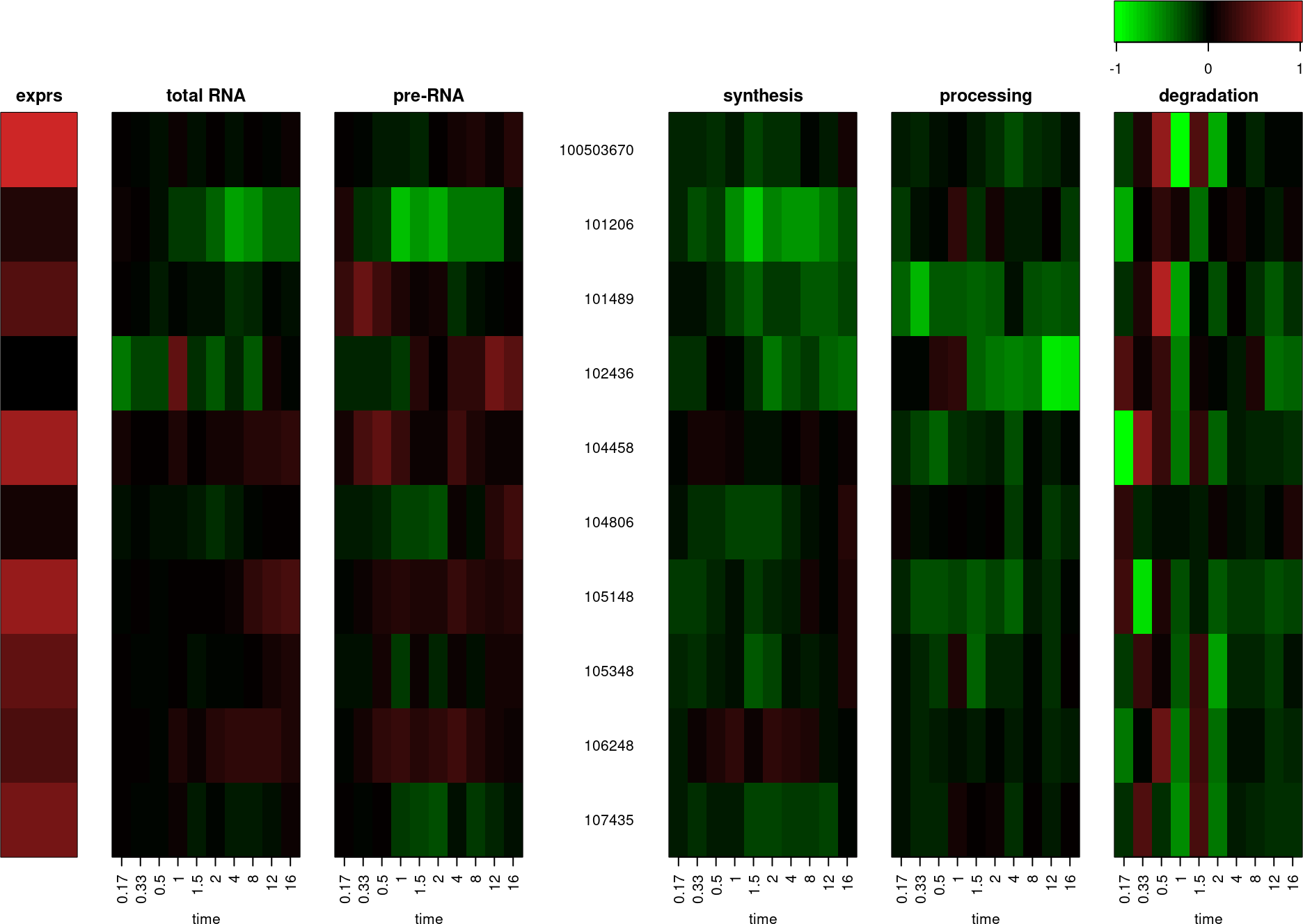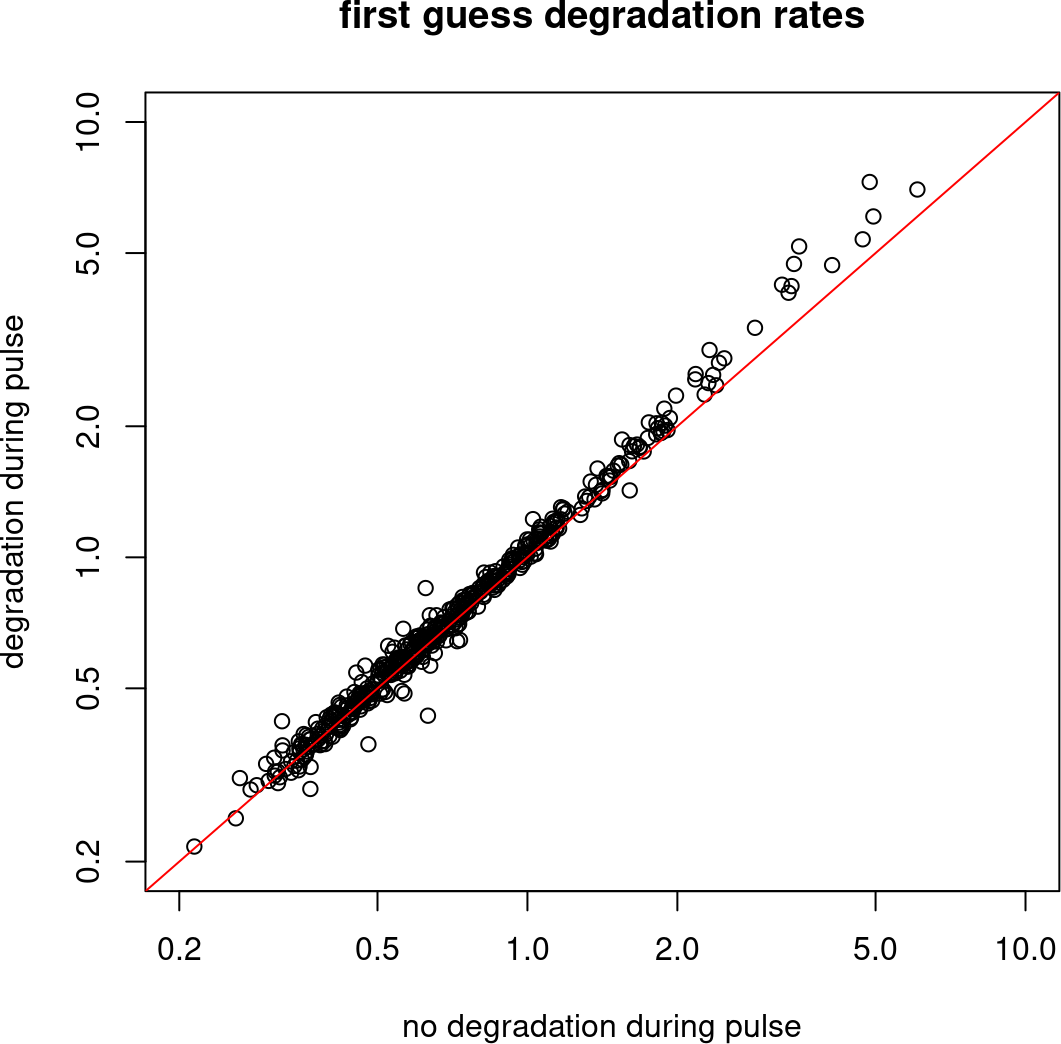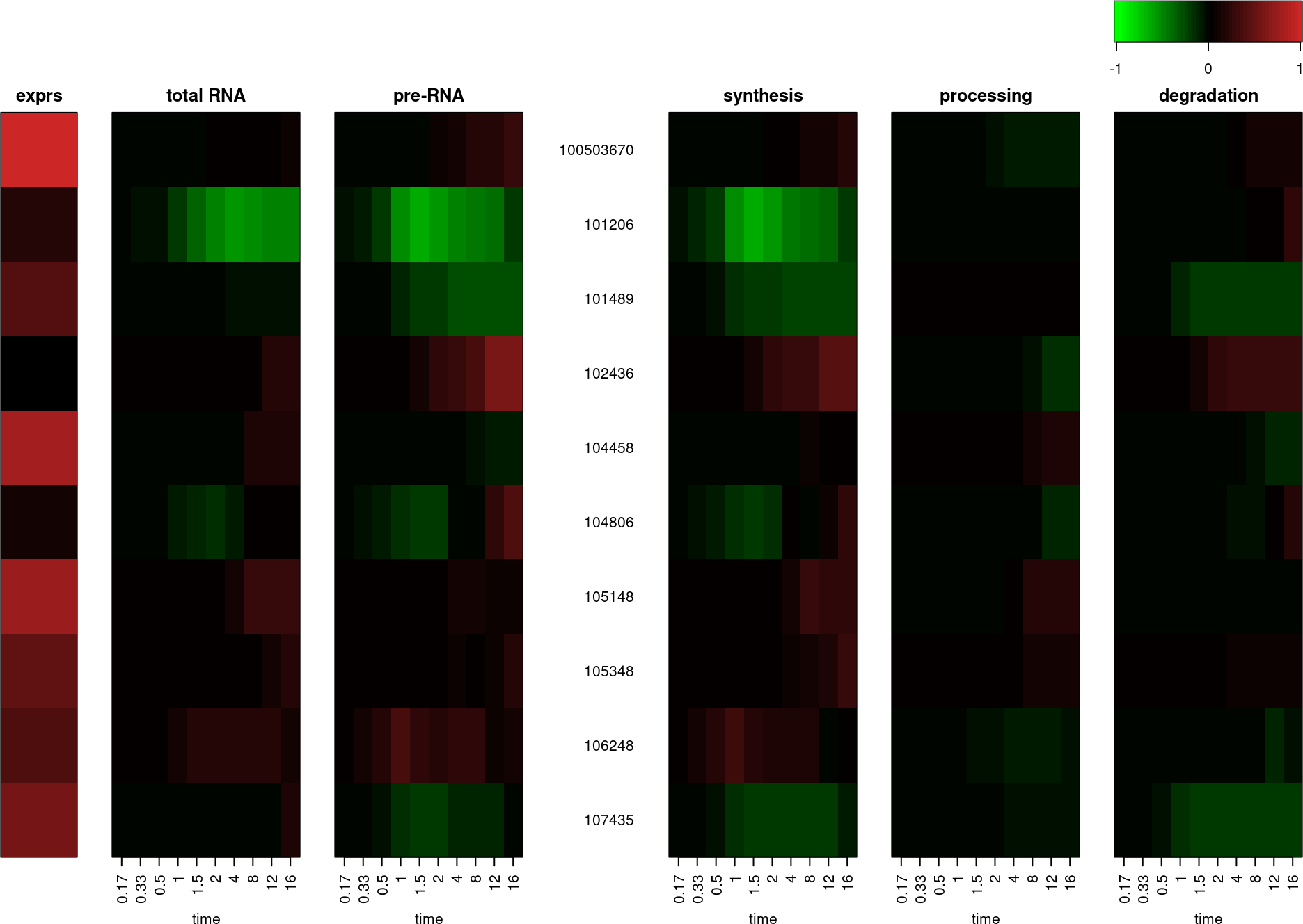# 1 Introduction

INSPEcT is an R/Bioconductor compliant solution for the study of RNA transcriptional dynamics from RNA-seq data (De Pretis et al. 2015, @Furlan520155). It is based on a system of two ordinary differential equations (ODEs) that describes the synthesis ($$k_1$$) and processing ($$k_2$$) of premature RNA ($$P$$) and the degradation ($$k_3$$) of mature RNA ($$M=T-P$$):

$\begin{equation} \left\{ \begin{array}{l l} \dot{P}=k_1 - k_2 \, \cdot \, P \\ \dot{T}=k_1 - k_3 \, \cdot \, (T - P) \end{array} \right. \tag{1} \end{equation}$

The total RNA ($$T$$) is measured from the exonic quantification of transcripts in RNA-seq experiment, while premature RNA ($$P$$) is measured by the intronic quantification. The model is based on two commonly accepted assumptions: nuclear degradation is deniable, and nuclear export occurs at a rate considerably faster than other rates and can be disregarded.

The package supports the analysis of several experimental designs, such as steady-state conditions or time-course data, and the presence or absence of the nascent RNA library. According to the experimental design, the software returns different outputs. In particular, when the nascent RNA is present (INSPEcT+), the rates of synthesis, processing and degradation can be calculated both in time-course and in steady-state state conditions and tested for differential regulation. Without the information from the nascent RNA (INSPEcT-), the rates of synthesis, processing and degradation, as well as their significant variation, can be calculated only in time-course, while only a variation in the ratio between processing and degradation (post-transcriptional ratio) can be assessed between steady states. A schema of the possible configurations and related outputs is reported in Table 1 and 2.

Table 1: INSPEcT+ experimental design
Time-course Yes Yes Yes

Table 2: INSPEcT- experimental design
Time-course Yes Yes Yes

Within the next sections, the usage of INSPEcT will be explained in detail. In particular, section 2 explains the different method to quantify intronic and exonic features from different input sources (alignments, read counts or direct quantifications) and create the input for the following INSPEcT analysis. Section 3 explains how to perform time-course analyses and visualize results. Section 4 explains how to perform steady-state analyses and visualize results. Finally, section 5 introduces two wrapper functions, which allow the user running the entire INSPEcT pipeline with a single command line.

Please also refer to the INSPEcT-GUI vignette. This graphic-user-interface exploits the key INSPEcT functionalities and facilitates the understanding of the impact of RNA kinetic rates on the dynamics of premature and mature RNA.

# 2 Quantification of Exon and Intron features

The INSPEcT analysis starts with the quantification of exonic and intronic quantifications and the estimation of their variance from the replicates in the different conditions of the analysis. INSPEcT can estimate transcripts abundances and associated variances starting from different entry points according to the source of data available: SAM (or BAM) files, read counts or transcripts abundances. The user can choose to estimate the variance by means of two different softwares: DESeq2 or plgem. By default, DESeq2 is used when starting from BAM or read counts, while plgem is used when starting from transcripts abundances.

library(INSPEcT)

## 2.1 From BAM or SAM files

INSPEcT can quantify transcripts abundnaces and variances directly from SAM or BAM files. In this case, transcripts annotations are retrieved from a TxDb object. By default, INSPEcT collapses the exons of the transcripts that belong to the same gene (argument by=“gene”). Alternatively, it can work also at the transcript level (argument by=’tx). When working at the transcript level, INSPEcT cannot discriminate between transcript, therefore we suggest assigning reads to all the overlapping features by setting the argument allowMultiOverlap to TRUE. INSPEcT manage strandness of the reads with the argument strandSpecific, which can be set to 0 in case of unstranded reads, 1 for stranded and 2 for reverse-stranded experiments. INSPEcT prioritizes the exon annotation, meaning that only the reads that do not overlap with any of the annotated exon are eventually accounted for introns. Here we report an example where the data from 4 sample BAM files (2 time points, 2 replicates each, as specified with the argument experimentalDesign) is quantified in a non-stranded specific way, at the gene level, not assigning the reads mapping to more than one feature.

require(TxDb.Mmusculus.UCSC.mm9.knownGene)
txdb <- TxDb.Mmusculus.UCSC.mm9.knownGene

bamfiles_paths <- system.file('extdata/',
c('bamRep1.bam','bamRep2.bam','bamRep3.bam','bamRep4.bam'),
package='INSPEcT')

exprFromBAM <- quantifyExpressionsFromBAMs(txdb=txdb
, BAMfiles=bamfiles_paths
, by = 'gene'
, allowMultiOverlap = FALSE
, strandSpecific = 0
, experimentalDesign=c(0,0,1,1))

The function returns a list containing exonic/intronic expressions and variances, as well as exonic/intronic feature widths extracted from the annotation, exonic/intronic counts and counts statistics.

names(exprFromBAM)
##  "exonsExpressions"   "intronsExpressions" "exonsVariance"
##  "intronsVariance"    "exonsWidths"        "intronsWidths"
##  "exonsCounts"        "intronsCounts"      "countsStats"
matureCounts<-allcounts$mature expDes<-rep(c(0,1/6,1/3,1/2,1,1.5,2,4,8,12,16),3) nasExp_DESeq2<-quantifyExpressionsFromTrCounts( allcounts=nascentCounts ,libsize=nascentLS ,exonsWidths=exWdths ,intronsWidths=intWdths ,experimentalDesign=expDes) matExp_DESeq2<-quantifyExpressionsFromTrCounts( allcounts=matureCounts ,libsize=totalLS ,exonsWidths=exWdths ,intronsWidths=intWdths ,experimentalDesign=expDes) ## 2.3 From transcripts abundances In order to exemplify the quantification of mean transcripts abundances and variances starting from their replicated quantification, we transform the read counts loaded from the package in the section above into RPKMs using the width of introns and exons and the library sizes. trAbundancesFromCounts <- function(counts, widths, libsize) t(t(counts/widths)/libsize*10^9) nascentTrAbundance <- list( exonsAbundances=trAbundancesFromCounts( nascentCounts$exonsCounts, exWdths, nascentLS),
intronsAbundances=trAbundancesFromCounts(
nascentCounts\$intronsCounts, intWdths, nascentLS))

Once we have obtained transcripts abundances for introns and exons in the different conditions and replicates of the experimental design, we can calculate the mean expression and variance (using plgem) by:

nasExp_plgem<-quantifyExpressionsFromTrAbundance(
trAbundaces = nascentTrAbundance
, experimentalDesign = expDes)

# 3 Analysis of RNA dynamics in time-course experiments

## 3.1 Analysis of Total and Nascent RNA (INSPEcT+)

In case of the joint analysis of total and nascent RNA data, for each transcript with at least one intron, the exonic and intronic quantifications are available in the Total and in the Nascent RNA libraries. The system of equations (1) describes the dynamics of the total RNA population, but when integrated between zero and the time of labeling ($$t_L$$) it can be used to describe nascent RNA. For matter of simplicity INSPEcT assumes that, during the labeling time, synthesis and processing rates are constant and nascent transcripts are not degraded. The set of equations to describe Total and Nascent RNA is:

$\begin{equation} \left\{ \begin{array}{l l} \dot{P}_{R}=k_1 - k_2 \, \cdot \, P_{R} \\ \dot{T}_{R}=k_1 - k_3 \, \cdot \, (T_{R} - P_{R}) \\ s_F \, \cdot \, P_{L}=\frac{k_1}{k_2} - ( 1 - e^{k_2 \, \cdot \, t_L} ) \\ s_F \, \cdot \, T_{L}=k_1 \, \cdot \, t_L \end{array} \right. \tag{2} \end{equation}$

where $$P_{R}$$ and $$T_{R}$$ are the premature and total RNA levels, respectively, estimated from the total RNA library, $$P_{L}$$ and $$T_{L}$$ are premature and total RNA levels, respectively, estimated from the nascent RNA library at each condition or time-point. First of all, $$\dot{P}_{R}$$ and $$\dot{T}_{R}$$ are estimated from the interpolation of $$P_R(t)$$ and $$T_R(t)$$ via cubic splines. INSPEcT exploits the overdetermination of the system (three unknowns: $$k_1$$, $$k_2$$ and $$k_3$$; and four equations) to calculate a scaling factor ($$s_F$$) between the Total and Nascent RNA for each condition or time-point (De Pretis et al. 2015).

### 3.1.1 First guess estimation of the rates

The rates of synthesis, processing and degradation are calculated from the Total and Nascent RNA quantifications by the newINSPEcT function:

  tpts<-c(0,1/6,1/3,1/2,1,1.5,2,4,8,12,16)
tL<-1/6
nascentInspObj<-newINSPEcT(tpts=tpts
,labeling_time=tL
,nascentExpressions=nasExp_DESeq2
,matureExpressions=matExp_DESeq2)

During this step, INSPEcT calculates a scaling factor between each time-point of Total and Nascent expressions (as described in the section above) and integrates the differential equations (2) assuming that, between experimental observations, total RNA, premature RNA and synthesis rate, behave linearly (linear piecewise), and that processing and degradation rates are constant (constant piecewise). Rates estimated by this procedure can be accessed from the INSPEcT object by the method ratesFirstGuess, which allows to access also RNA concentrations and variances associated to the experimental observations:

  round(ratesFirstGuess(nascentInspObj,'total')[1:5,1:3],3)
##           total_0 total_0.166666667 total_0.333333333
## 100503670 605.805           609.366           601.865
## 101206      5.107             5.356             5.196
## 101489     15.629            15.767            15.606
## 102436      1.543             1.107             1.305
## 104458     86.966            93.632            88.837
  round(ratesFirstGuessVar(nascentInspObj,'total')[1:5,1:3],3)
##           total_0 total_0.166666667 total_0.333333333
## 100503670 775.569           784.570           764.586
## 101206      0.382             0.417             0.392
## 101489      1.192             1.208             1.179
## 102436      0.281             0.145             0.202
## 104458     24.917            28.736            25.866
  round(ratesFirstGuess(nascentInspObj,'synthesis')[1:5,1:3],3)
##           synthesis_0 synthesis_0.166666667 synthesis_0.333333333
## 100503670     231.119               211.859               210.099
## 101206          4.875                 4.412                 3.964
## 101489          7.749                 7.412                 7.421
## 102436         32.087                28.312                28.645
## 104458         47.333                46.424                50.867

The INSPEcT object can be subset to focus on a specific set of genes, and for the sake of speeding up the downstream analysis, we will focus on the first 10 genes of the INSPEcT object.

nascentInspObj10<-nascentInspObj[1:10]

The complete pattern of total and pre-mRNA concentrations variation together with the synthesis, degradation and processing rates can be visualized using the inHeatmap method (Figure 1):

inHeatmap(nascentInspObj10, clustering=FALSE)Figure 1: inHeatmap of the ratesFirstGuess
Heatmap representing the concentrations of total RNA, of premature RNA and the first guess of the rates of the RNA life cycle

In case of a long $$t_L$$ (more than 30 minutes), it can be useful to set the argument degDuringPulse equal to TRUE to estimate the rates of the RNA life-cycle without assuming that nascent transcripts are not degraded during the labeling time. The longer the labelling time is the weaker this assumption gets, however, taking into account nascent RNA degradation involves the solution of a more complicated system of equations and can originate unrealistic high rates of synthesis, processing and degradation.

  nascentInspObjDDP<-newINSPEcT(tpts=tpts
,labeling_time=tL
,nascentExpressions=nasExp_DESeq2
,matureExpressions=matExp_DESeq2
,degDuringPulse=TRUE)

For short labeling times, as 10 minutes can be considered, the absence of degradation during the pulse is a good assumption and similar rates are estimated in both cases. In Figure 2 we compared the degradation rates estimated with or without the assumption that degradation of mature RNA occurs during the labeling time.

k3 <- ratesFirstGuess(nascentInspObj, 'degradation')
plot(rowMeans(k3), rowMeans(k3ddp), log='xy',
xlim=c(.2,10), ylim=c(.2,10),
abline(0,1,col='red')Figure 2: Dotplot of degradation rates calculated with or without the assumption of no degradation during pulse
First guess of the degradation rates was calculated with or without degDuringPulse option. In red is represented the line of equation y = x.

### 3.1.2 Modeling with sigmoid and impulse functions

The rates evaluated during the “first guess” are highly exposed to the experimental noise. In particular, processing and degradation rates sum up the noise coming from different experimental data (i.e. the Total and the Nascent libraries), and could be challenging to distinguish real variations from noise. For this reason, the method modelRates fits either sigmoid or impulse functions to total RNA, processing and degradation rates, that minimize the error on experimental data. In this approach, premature RNA and synthesis rate functional forms directly result from the system of equations (1).

nascentInspObj10<-modelRates(nascentInspObj10, seed=1)

The rates computed through this modeling procedure are accessible via the method viewModelRates and can visualized via the method inHeatmap, setting the argument type=‘model’} (Figure 3).

round(viewModelRates(nascentInspObj10, 'synthesis')[1:5,1:3],3)
##           synthesis_0 synthesis_0.17 synthesis_0.33
## 100503670     208.688        208.688        208.688
## 101206          4.640          4.466          4.242
## 101489          7.524          7.454          7.348
## 102436         25.632         25.663         25.712
## 104458         47.738         47.738         47.738
inHeatmap(nascentInspObj10, type='model', clustering=FALSE)Figure 3: inHeatmap of the rates after the second step of modeling
Heatmap representing the concentrations of total RNA, of premature RNA and the rates of the RNA life cycle after the second step of modeling.

### 3.1.3 Confidence intervals estimation and selection of the regulatory scenario

For each rate and time point, 95% confidence intervals are calculated and used to test the variability of a rate. Specifically, a chi-squared goodness-of-fit test is performed to assess how a constant rate fit within confidence intervals. Confidence intervals can be accessed by the method viewConfidenceIntervals, the p-values of the rates variability tests can be accessed by the method ratePvals and the regulatory class assigned to each gene by the method geneClass. In particular, each gene is assigned to a class named after the set of varying rates: “no-reg” denotes a gene whose rates are constant over time, “s” denotes a gene whose synthesis changes over time, “p” denotes a gene whose processing changes over time, “d” denotes a gene whose degradation changes over time. Genes regualted by a more than one rate have a class composed by more letters, for example, the class “sp” represnents genes regulated at the level of both synthesis and processing rates.

head(ratePvals(nascentInspObj10),3)
##              synthesis processing degradation
## 100503670 8.663546e-01          1   0.9999919
## 101206    7.002705e-08          1   0.9999919
## 101489    1.612946e-03          1   0.0142093
head(geneClass(nascentInspObj10),3)
## 100503670    101206    101489
##  "no-reg"       "s"      "sd"

The method plotGene can be used to fully investigate the profiles of RNA concentrations and rates of a single gene. Estimated synthesis, degradation and processing rates, premature RNA and total RNA concentrations are displayed with solid thin lines, while their standard deviations or confidence intervals are in dashed lines and the modelled rates and concentrations are in thick solid lines. This example shows a gene of class “a”, indicating that its expression levels are controlled just by synthesis rate (Figure 4).

plotGene(nascentInspObj10, ix="101206")